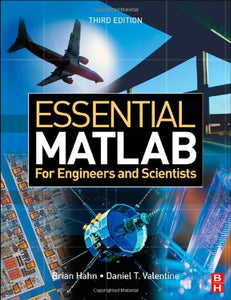Brand: Newnes

# Essential MATLAB for Engineers and Scientists, Third Edition

• Publish Date: 2007-03-22
• Binding: Paperback
• Author: Brian Hahn;Dan Valentine

Regular price \$14.96 Sale price \$119.97

Attention: For textbook, access codes and supplements are not guaranteed with used items.

The essential guide to MATLAB as a problem solving tool

This text presents MATLAB both as a mathematical tool and a programming language, giving a concise and easy to master introduction to its potential and power. Stressing the importance of a structured approach to problem solving, the text gives a step-by-step method for program design and algorithm development. The fundamentals of MATLAB are illustrated throughout with many examples from a wide range of familiar scientific and engineering areas, as well as from everyday life.

Features:

. Numerous simple exercises provide hands-on learning of MATLAB's functions
. A new chapter on dynamical systems shows how a structured approach is used to solve more complex problems.
. Common errors and pitfalls highlighted
. Concise introduction to useful topics for solving problems in later engineering and science courses: vectors as arrays, arrays of characters, GUIs, advanced graphics, simulation and numerical methods
. Text and graphics in four colour
. Extensive instructor support

Essential MATLAB for Engineers and Scientists is an ideal textbook for a first course on MATLAB or an engineering problem solving course using MATLAB, as well as a self-learning tutorial for students and professionals expected to learn and apply MATLAB for themselves.

Additional material is available for lecturers only at http://textbooks.elsevier.com. This website provides lecturers with:

• A series of Powerpoint presentations to assist lecture preparation
• Extra quiz questions and problems
• M-files for the exercises and examples in the text (also available to students at the book's companion site)
• Solutions to exercises
• An interview with the revising author, Daniel Valentine

Numerous simple exercises give hands-on learning
A chapter on algorithm development and program design
Common errors and pitfalls highlighted
Concise introduction to useful topics for solving problems in later engineering and science courses: vectors as arrays, arrays of characters, GUIs, advanced graphics, simulation and numerical methods
A new chapter on dynamical systems shows how a structured approach is used to solve more complex problems.
Text and graphics in four colour
Extensive teacher support on http://textbooks.elsevier.com: solutions manual, extra problems, multiple choice questions, PowerPoint slides
Companion website for students providing M-files used within the book